# Volume Of A Cuboid Worksheet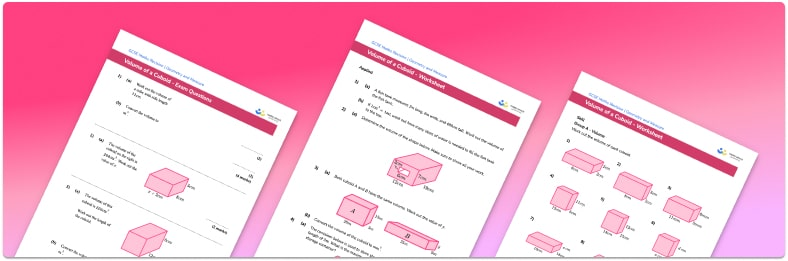• Section 1 of the volume of a cuboid worksheet contains 27 skills-based volume of a cuboid questions, in 3 groups to support differentiation
• Section 2 contains 4 applied volume of a cuboid questions with a mix of word problems and deeper problem solving questions
• Section 3 contains 3 foundation and higher level GCSE exam style volume of a cuboid questions
• Answers and a mark scheme for all volume of a cuboid questions are provided
• Questions follow variation theory with plenty of opportunities for students to work independently at their own level
• All questions created by fully qualified expert secondary maths teachers

Suitable for GCSE maths revision for AQA, OCR and Edexcel exam boards

• This field is for validation purposes and should be left unchanged.

You can unsubscribe at any time (each email we send will contain an easy way to unsubscribe). To find out more about how we use your data, see our privacy policy.

### Volume of a cuboid at a glance

Volume is a measure of the amount of space inside a 3D shape. To find the volume of a cuboid or the volume of a cube, we can use the formula Volume =L \times W \times H where L is the length of the cuboid, W is the width of the cuboid and H is the height of the cuboid.

The volume of the cuboid is measured in cubic units such as cubic centimetres (cm^3) or cubic metres (m^3).

We can find missing lengths of cuboids given the cuboid volume and some side lengths. To do this, we substitute the values we know into the volume formula and solve to find the missing values.

Looking forward, students can then progress to additional volume worksheets and other geometry worksheets, for example a simplifying expressions worksheet or simultaneous equations worksheet.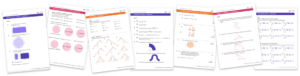For more teaching and learning support on Geometry our GCSE maths lessons provide step by step support for all GCSE maths concepts.

## Related worksheets

Volume Of A Cone Worksheet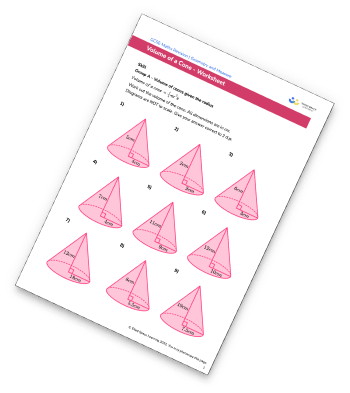Volume Of A Cylinder Worksheet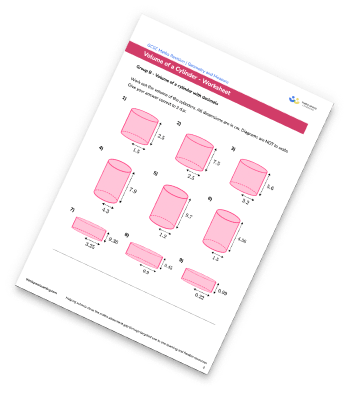Congruent Triangles Worksheet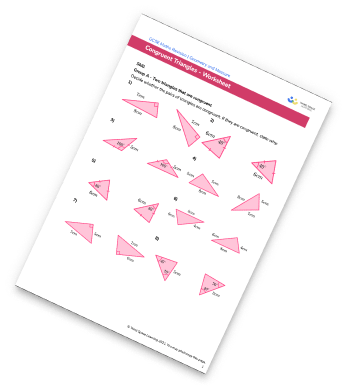Surface Area Of A Triangular Prism Worksheet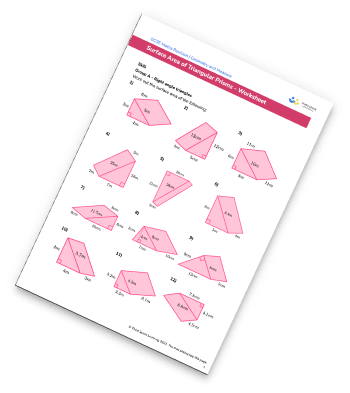## Do you have KS4 students who need more focused attention to succeed at GCSE?There will be students in your class who require individual attention to help them succeed in their maths GCSEs. In a class of 30, it’s not always easy to provide.

Help your students feel confident with exam-style questions and the strategies they’ll need to answer them correctly with our dedicated GCSE maths revision programme.

Lessons are selected to provide support where each student needs it most, and specially-trained GCSE maths tutors adapt the pitch and pace of each lesson. This ensures a personalised revision programme that raises grades and boosts confidence.

Find out more